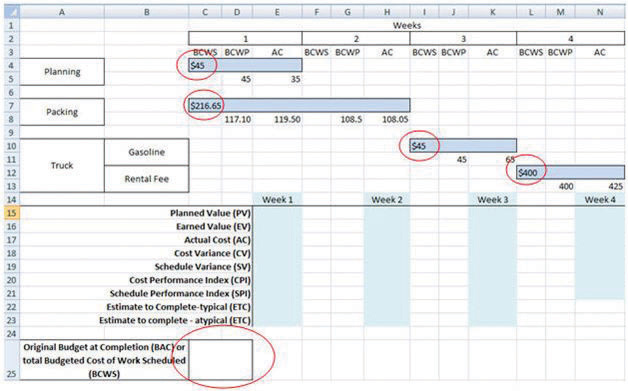# 9.3: Exercises

Exercises at the end of the chapter are designed to strengthen your understanding and retention of the information recently acquired in the chapter.

The questions in this section refer to the project budget shown below.

Figure 9.10 Example for Short Answer QuestionsThis project has three completed activities whose durations are indicated by the blue bars in row 2. The budgeted cost of work scheduled for each task is shown on the bar in row 2. The budgeted cost of work scheduled for each week is shown on row 3. Notice that the amounts in row 3 add up to the amounts shown on the bars. Rows 4 and 5 indicate the budgeted cost of work performed and actual cost. Notice that the second task started a week late.

1. What is the planned value (PV) at the end of week three?
2. What is the earned value (EV) at the end of week three?
3. What is the actual cost (AC) at the end of week three?
4. What is the cost variance (CV) at the end of week three?
5. What is the schedule variance (SV) at the end of week three?
6. What is the planned value (PV) at the end of week seven?
7. What is the earned value (EV) at the end of week seven?
8. What is the actual cost (AC) at the end of week seven?
9. What is the cost variance (CV) at the end of week seven?
10. What is the schedule variance (SV) at the end of week seven?
11. What is the cost performance index (CPI) at the end of week seven?
12. What is the schedule performance Index at the end of week seven?
13. If the budget at completion (BAC) for this project is $500,000, what is the Estimate to Complete (ETC) at the end of week seven if the cost and schedule variances experienced are typical of the remainder of the activities? 14. Based on your answer to the previous question, what is the estimate at completion (EAC) for this project? ## Essay Questions Write several paragraphs to provide more in-depth analysis and consideration when answering the following questions. 1. A project is about a third of the way through its scheduled activities, and an earned value management report has been prepared. The summary indicates that it has a CPI of 0.9 and an SPI of 0.8. What do these two values tell you about the project? 2. A project is about half done with a CV of$(4,000) due to atypical costs in the first half of the project. Its project manager provides an ETC of \$50,000. Interpret this statement in your own words.
3. Give an example of an analogous estimate and a parametric estimate that are different from those described in the text.

## Discussion

The exercises in this section are designed to promote exchange of information among students in the classroom or in an online discussion. The exercises are more open ended, which means that what you find might be completely different from what your classmates find, and you can all benefit by sharing what you have learned.

1. Describe an experience of your own that involved a parametric estimate. Describe the parameter and how it was used. Compare the estimate with the actual cost.
2. As an inexperienced project manager, how do you develop expert knowledge? Interview someone who would qualify as an expert in estimating costs and ask them to describe the process they went through. Ask them for advice on how to become an acknowledged expert in estimating and then share what you learn with your classmates.